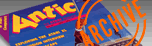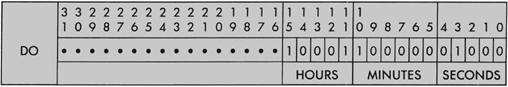`ANTIC VOL. 6, NO. 7 / NOVEMBER 1987`

# Tap the Power of Your System Clock

### Improve your timing with ST BASIC

By STEPHEN ORIOLD

A few weeks ago I wrote a set of benchmarks to test a variety of personal computers, including the ATARI ST The benchmarks (integer math, real math, logarithmic and trigonometric functions, screen output speed and disk I/O speed) had to be written in BASIC, the language included free with every PC I tested.

As I was writing the benchmarks, the time came when I had to access the system clock from BASIC, and on my own ST, I found out that I couldn't. Well, after reading everything I could get my hands on about the system clock, I discovered that, although there seem to be two clocks running in the ST simultaneously, there is no way to directly access either one of them from ST BASIC.

Okay then, we'll do it in assembly language. This is the basic idea:

• write an assembly language routine to read the system clock, decode the time, and store it in memory
• assemble the routine
• write a BASIC program, incorporating the machine language instructions (assembler output) to access the memory locations containing the time, read the time and display it.

And that’s it. Sounds fairly easy, doesn't it? Well, it's not quite that easy, and here's why. GEMDOS offers two function calls to access the clock: \$2C (GET TIME) and \$2D (SET TIME). The GET TIME call requires no parameters, and returns the time in the low word of the 68000's data register D0.

Unfortunately, it's so well-encoded that it'll make your life miserable. This GEMDOS clock starts running immediately at start-up (whether you set it first or not), although it might not have the right time, using instead the time preset by ATARI. The GET TIME call returns the hour of the day, minutes and seconds. GEMDOS uses different calls (\$2A, GET DATE and \$2B, SET DATE) to access the system date.

There are other ways to access the clock: through IKBD (intelligent keyboard) commands \$1B (Set clock) and \$1C (Read clock), or XBIOS calls 22 (settime) and 23 (gettime). Both the IKBD and the XBIOS call return both the date and the time of day. The IKBD call returns this information in packed BCD, in six bytes and a time of day event header. The XBIOS gettime call requires no parameters and returns the date and time, encoded, in a longword, with the time in the low order word.

I decided to use the GEMDOS call for my application. Let's take a closer look at this GEMDOS time. The GET TIME call returns the time in register DO, encoded in a special pattern of individual bits. Take a look at Figure 1 and you'll see what I mean.`Figure 1`

The number of seconds is stored in bits O-4 (five bits). Since the maximum number you can represent in five bits is 31, the GEMDOS clock runs in two-second increments. To get the correct number of seconds, the value stored in these five bits has to be multiplied by two.

The number of minutes is stored in bits 5-10 (six bits), the number of hours, in 24-hour format, in bits 11-15 (five bits). In the above example, the value of hours is 17 (5 pm), the value of minutes is 32, and the value of seconds is 8, translating to 16 seconds (17:32:16).

I wrote TIME.S, the assembly language source file in Listing 1, to read, decode and store the GEMDOS time. It was assembled using DRI'S AS68, on an upgraded one-megabyte 520ST.

Here is what this program is doing:

```  Line l-save assembly language routine address in Al.
Line 2--push GEMDOS function call number on stack
Line 3--execute function call
Line 4--repair stack
Line 5--move time bit pattern to Dl
Line 6--keep bits representing seconds, set everything else to zeroes
Line 7--multiply number of seconds by 2
Line 8--save bit pattern representing seconds in memory, in the first word immediately following the assembly language routine
Line 9--shift bit pattern 5 positions to the right, truncating bits representing seconds, shift zeroes into high-order bits
Line 10-move resulting bit pattern, representing minutes and hours into the second word in memory, following the assembly language routine
Line 11-keep bits representing minutes, set everything else to zeroes
Line 12-shift bit pattern 6 positions to the right, truncating bits representing minutes, shift zeroes into high order bits
Line 13-move resulting bit pattern, representing now only the number of hours, into the third	available word in memory.
Line 14-return control to the program that issued the CALL.```

That was the assembly language part. Now comes the BASIC program in Listing 2. This will create an integer array, consisting of the opcodes output by the assembler, found in the data statements. The 0th element of the array will contain the address of the machine language program, elements 21, 22 and 23 the seconds, minutes and hours. The program will read the address in element 0, then CALL the machine language routine, create a nice string containing the time data and output it.

If you intend to use this BASIC program as a subroutine in your own programs, move line 1040 to the initialization module of your own program, delete lines 1060-1080, 1320-1330 and all the REMarks, especially the one in line, 1400.

This is not the only, probably not even the best way to, access the system clock from ST BASIC. But it's simple, short, fast enough (for ST BASIC) and it can get the job done, until a better BASIC comes along.

## USING GFA BASIC

Sometimes, you have to do radically different things to get the same job done in another version of the same language. Take, for example, GFA BASIC. Here, no machine language help is needed. GFA gives almost full access to the complete power of your Atari ST.

Examine Listing 3. This is the same program, written in GFA BASIC, but also show how to set the time and date as well as access them. Of course, getting the Time and Date from GFA is as easy as stating: T\$=TIME\$, or D\$=DATE\$. Actually setting the time, however, is a bit more involved. Let's investigate how this is done.

#### Get_the_date

This routine first allows the user to type in the date in the typical format used in North America, MM/DD/YYYY. The interesting line here is:

Let Datein%=(Year*512)+(Month*32)+(Day).

After we've determined we have the proper numbers for the year, month and day we use multiplication to "left-shift” the bit-patterns for the date numbers into their proper positions, and then add them together into a single, four-byte long number, suitable for passing to the proper Gemdos( ) routine.

#### Handle_d_key

This procedure will collect keystrokes into the format of the date we want.

#### Get-the-time

The routine operates in essentially the same manner as the date routine. The proper digits are collected into a string, which is then taken apart to acquire the proper digits for passing to the Gemdos routine to set the time.

Finally, when the Time and Date have been grabbed and set, the programs returns to the top, where a small Alert Box is built up out of the current Time\$ and Date\$, and the results are reported. And that’s all there is to it! I hope these simple BASIC routines can help you read your ST’s internal clock, and that I've come to your aid-in time.

Listing 1```1000 ' 1010 GETTIME : 1020 '(c) 1987 Antic Publishing 1030 'Version 010887 1040 'Written by Stephen Oriold 1050 ' 1060 Dim OPCODE%(23) 1070 ' 1060 ' 1090 restore 1340 1100 ' 1110 for C%=0 to 23 1120 read OPCODE%(C%) 1130 next C% 1140 ' 1150 ADDR=varptr(OPCODE%(0)) 1160 call ADDR 1170 ' 1180 HRS%=OPCODE%(23) 1190 MINX=OPCODE%(22) 1200 SEC%=OPCODE%(21) 1210 1220 HRS\$=str\$(HRS%) 1230 if len(HRS\$)=2 then HRS\$=" 0" + right\$(HRS\$, 1) 1240 MIN\$=str\$(MIN%) 1250 if len(MIN\$)=2 then MIN\$=" 0" + right\$(MIN\$, 1) 1260 SEC\$=str\$(SEC%) 1270 if len(SEC\$)=2 then SEC\$=" 0" + right\$(SEC\$, 1) 1280 ' 1290 TIMES=right\$(HRS\$, 2) + "." + right\$(MIN\$, 2) 1300 TIMES=TIME\$ + ":" + right\$(SEC\$, 2) 1310 print TIME\$ 1320 input "- Hit [RETURN] to exit.", AS 1330 ' 1340 data &H2248, &H3F3C, &H002C, &H4E41, &H548F, &H3200 1350 data -15748, &H001F, -7351, &H3341, &h002A, -5560 1360 data &H3340, &H002C, &H0269, &H003F, &H002C, -5048 1370 data &H3340, &H002E, &H4E75, &H0000, &H0000, &H0000```

Listing 2

``CP/M 68000 Assembler  Revision 04.03 Page 1 Source File: B:TIME.S 1 00000000 2248		MOVE.L	A0, A1 2 00000002 3F3C002C		MOVE.W	#\$2C, -(A7) 3 00000006 4E41		TRAP	#1 4 00000000 548F		ADDQ.L	#2, A7 5 OOOOOOOA 3200		MOVE.W	D0, D1 6 OOOOOOOC C27C001F		AND.W	#%11111, D1 7 00000010 E349		LSL.W	#1, D1 8 00000012 3341002A		MOVE.W	D1, \$2A(A1) 9 00000016 EA48		LSR.W	#5, D010 00000018 3340002C		MOVE.W	D0, \$2C(A1)11 0000001C 0269003F002C	AND.W	#%111111, \$2C(A1)12 00000022 EC48		LSR.W	#6, D013 00000024 3340002E		MOVE.W	D0, \$2E(A1)14 00000028 4E75		RTS15``

Listing 3

``````'
' Time and Date Setting program
' (c) 1987 Antic Publishing
' version 072887
' Written by Patrick Bass
'
' - - - - - - - - - - - - - -
'
@Get_the_date
@Get_the_time
Print
Alert 0,"Time: "+Time\$+" |Date: "+Date\$+" | ",1,"Exit",Button
'
End
'
' ---------------------------
Procedure Get_the_date
Repeat
CLS
Print " Enter Today's Date"
'
Print At(2,3);" Date format: mm/dd/yyyy  (";Date\$;")"
Let Current_date\$=""
Print At(2,4);"Today's Date: ";
Let Selected=False
'
Repeat
@Handle_d_key
Until Selected
'
Let Month\$=Left\$(Current_date\$,2)
Let Day\$=Mid\$(Current_date\$,4,2)
Let Year\$=Right\$(Current_date\$,4)
'
If Month\$>="01" And Month\$<="12"
If Day\$>="01" And Day\$<="3l"
If Year\$>="1980" And Year\$<="2099"
Let Proper_date_format=True
Let Year=(Val(Year\$)-1980)
Let Month=(Val(Month\$))
Let Day=(Val(Day\$))
Let Datein%=(Year*512)+(Month*32)+Day
Let X=Gemdos(43,W:Datein%)
Endif
Endif
Endif
'
If Not Proper_date_format
Let Current_date\$=""
Let Dl=0
Endif
'
Until Proper_date_format
Return
' ------------------------------------
Procedure Handle_d_key
Let Keyd\$=Inkey\$
Let Selected=True
Endif
'
If Keyd\$>="0" And Keyd\$<="9"
If Len(Current_date\$)<10
Let Current_date\$=Current_date\$+Keyd\$
'
If Len(Current_date\$)=2 Or Len(Current_date\$)=5
Let Current_date\$=Current_date\$+"/"
Let Dl=Len(Current_date\$)
Endif
'
Endif
Print At(16,4);"                         ";
Print At(16,4);Current_date\$;
Endif
'
Return
'
' ----------------------------------
Procedure Get_the_time
Repeat
Cls
Print At(2,2);"Enter The Current Time in 24 Hour Format"
'
Print At(2,3);" Time format: hh:mm:ss  (";Time\$;")"
Let Current_time\$=""
Print At(2,4);"Current Time: ";
Let Selected=False
'
Repeat
@Handle_t_key
Until Selected
'
Let Hour\$=Left\$(current_time\$,2)
Let Minute\$=Mid\$(Current_time\$,4,2)
Let Second\$=Right\$(Current_time\$,2)
'
If Hour\$>="00" And Hour\$<="23"
If Minute\$>="00" And Minute\$<="59"
If Second\$>="00" And Second\$<="59"
Let Proper_time_format=True
Let Hours=(Val(Hour\$))
Let Minutes=(Val(Minute\$))
Let Seconds=(Val(Second\$))
Let Time_in%=(Hours*2048)+(Minutes*32)+(Seconds/2)
Let X=Gemdos(45,W:Time_in%)
Endif
Endif
Endif
'
If Not Proper_time_format
Let Current_time\$=""
Let Tl=0
Endif
'
Until Proper_time_format
Return
'
' ---------------------------------
Procedure Handle_t_key
'
Let Keyt\$=Inkey\$
If Keyt\$=Chr\$(13)
Let Selected=True
Endif
'
If Keyt\$>="0" And Keyt\$<="9"
If Len(Current_time\$)<8
Let Current_time\$=Current_time\$+Keyt\$
'
If Len(Current_time\$)=2 Or Len(Current_time\$)=5
Let Current_time\$=Current_time\$+":"
Let Tl=Len(Current_time\$)
Endif
'
Endif
Print At(16,4;"                          ";
Print At(16,4;Current_time\$;
Endif
'
Return
'
' -- End of program ---------------------``````